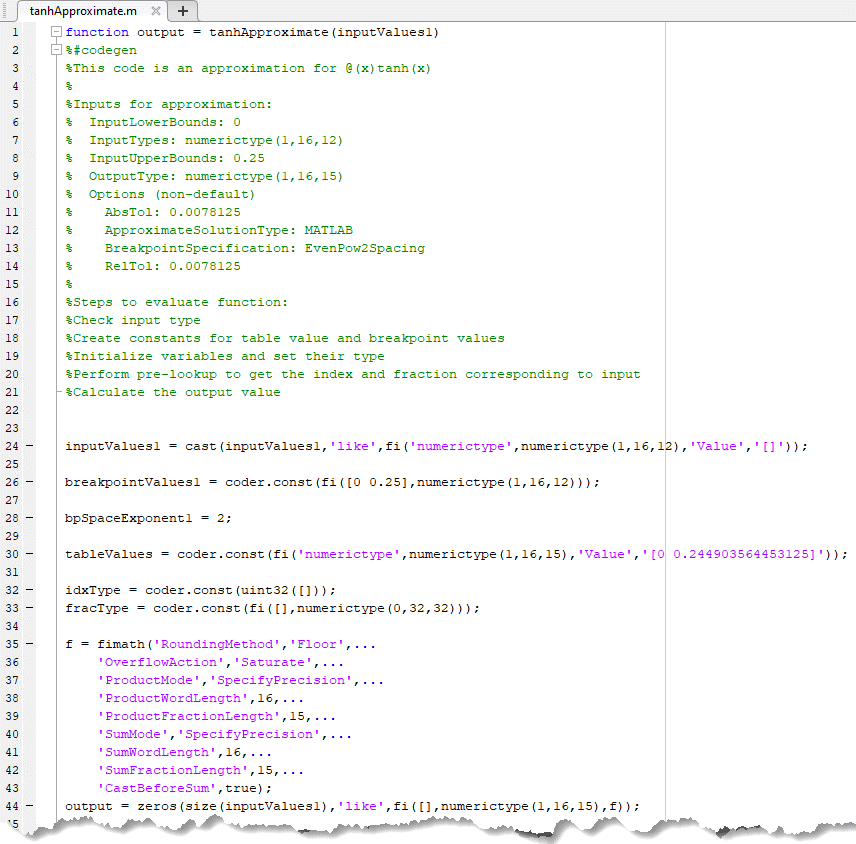Generate an Optimized Lookup Table as a MATLAB Function Programmatically

This example shows how to generate an optimized lookup table as a MATLAB® function to approximate hyperbolic tangent. The MATLAB function lookup table approximation can then be used to replace the hyperbolic tangent function and generate C code.

Use the FunctionApproximation.Options object to specify a MATLAB function as the solution type. Use the default values for accuracy and word length constraints.

options = FunctionApproximation.Options();
options.ApproximateSolutionType = 'MATLAB';

Specify the function to approximate and the input ranges and data types in the FunctionApproximation.Problem object.

functionToApproximate = 'tanh';

problem = FunctionApproximation.Problem(functionToApproximate, 'Options', options);
problem.InputLowerBounds = 0;
problem.InputUpperBounds = 0.25;

Use the solve method to solve the optimization problem and create a lookup table solution.

solution = solve(problem)
|  ID |  Memory (bits) | Feasible | Table Size | Breakpoints WLs | TableData WL | BreakpointSpecification |             Error(Max,Current) |
|   0 |             64 |        1 |          2 |              16 |           16 |             EvenSpacing |     7.812500e-03, 1.962196e-03 |
|   1 |             64 |        1 |          2 |              16 |           16 |         EvenPow2Spacing |     7.812500e-03, 1.962196e-03 |

Best Solution
|  ID |  Memory (bits) | Feasible | Table Size | Breakpoints WLs | TableData WL | BreakpointSpecification |             Error(Max,Current) |
|   1 |             64 |        1 |          2 |              16 |           16 |         EvenPow2Spacing |     7.812500e-03, 1.962196e-03 |
solution =
1x1 FunctionApproximation.LUTSolution with properties:

ID: 1
Feasible: "true"

To obtain the generated lookup table as a MATLAB function, use the approximate method. Use optional name-value arguments to specify the name and path for the lookup table function.

filename = 'tanhApproximate';
filepath = cd;
approximate(solution,'Name',filename,'Path',filepath);If you have MATLAB Coder™ installed, you can use the codegen command to generate C code from the approximate lookup table function.

inputArgs = linspace(1,10,10);
codegen tanhApproximate.m -args {inputArgs}
Code generation successful.